# Category theory

### April 26, 2020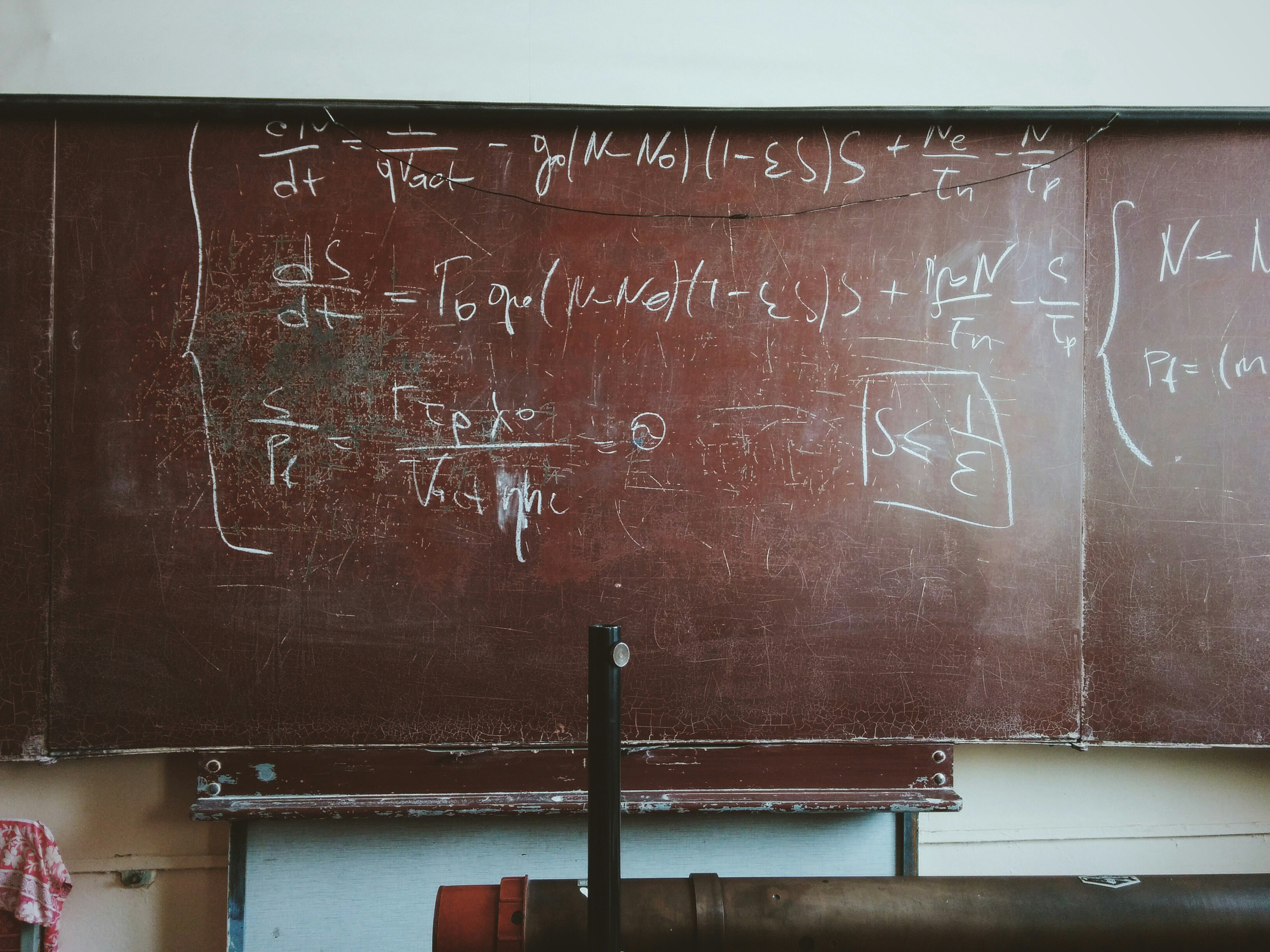Source## Disclaimer

This is short synopsis of great set of lectures. What is written here is by no means true, one should refer to original lectures or some books etc. This is written mostly for myself in case I wanted to revisit the topic in the future. Everything below is not “what it is” but mostly “how I understood that”. So, there might be mistakes and so on.

## Category

Category consists of:

• objects / dots (a, b, c, …)

• morphisims / arrows (f, g, …).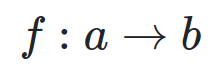morphism from a into b, connects two objects

• composition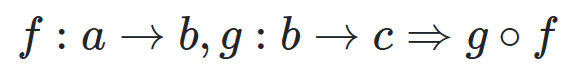apply g after f

And should:

• be associative: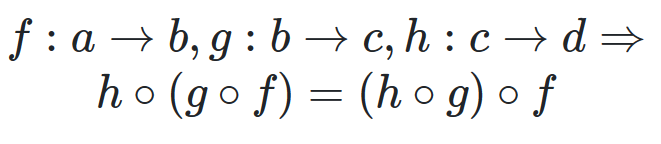Order of composition doesn’t matter

• have identity morphism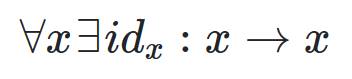There is an arrow to itself

## Category examples

• no objects

• no morphisms

### Category 1 (singleton)

• 1 object

• 1 identity morphism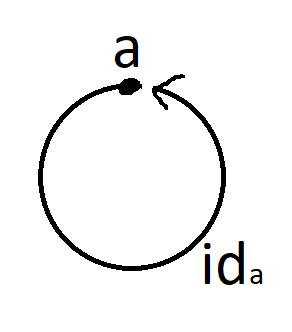### Category with 2 objects and 1 morphism

• 2 objects

• 1 morphism from one object to another

• 2 identity morphisms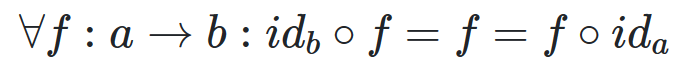Applying identity morphisms to any morphism is equivalent to that morphism itself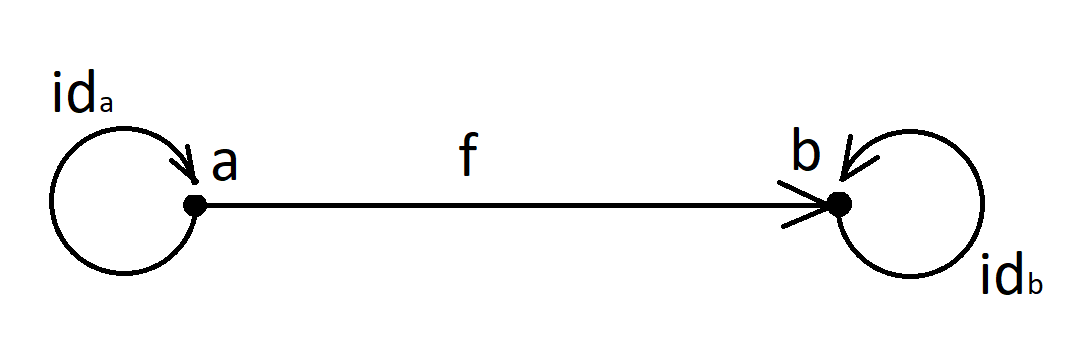## Universal construction

• identify pattern

• define ranking

• define best

## Iso- / mono- / epi- morphisms

### Isomorphismf is isomorfism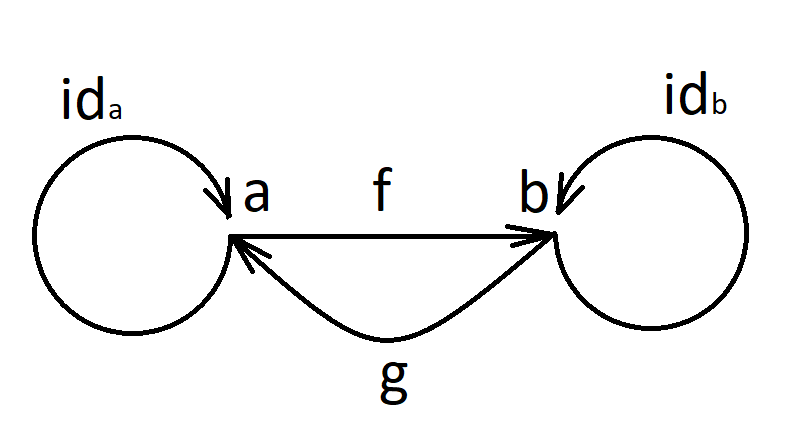Isomorphic implies inverse morphism

### Injective / monic / monomorphism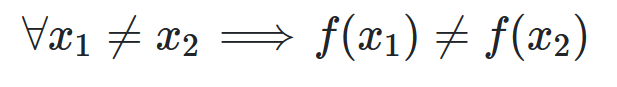f is monomorphism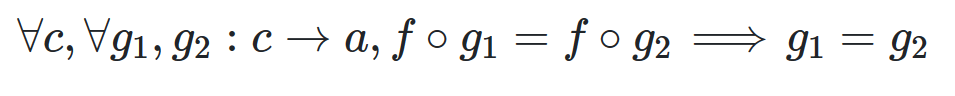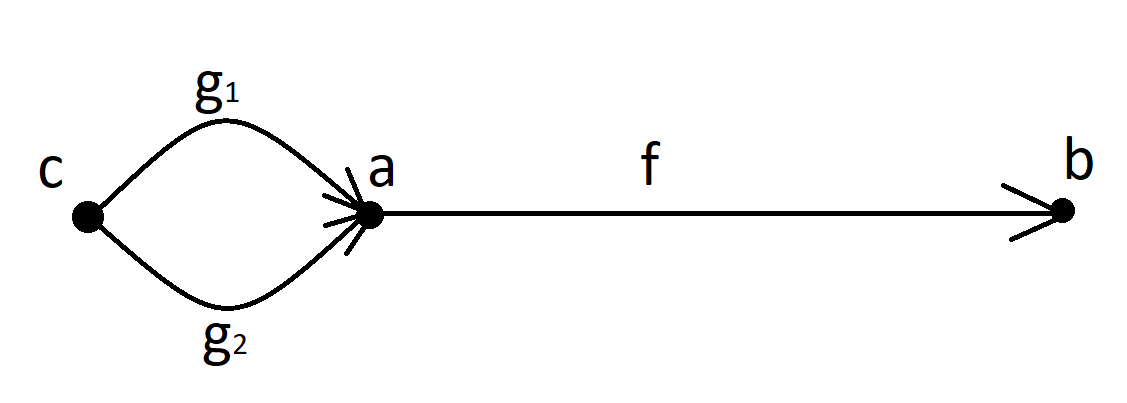### Surjective / epic / epimorphism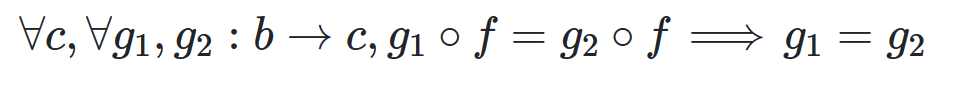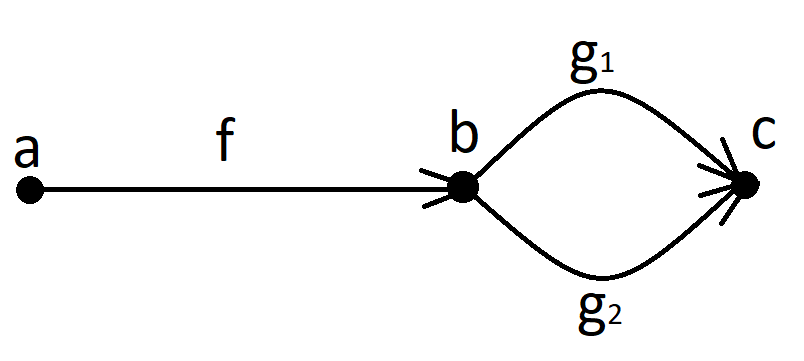## Order relation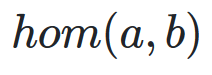Hom-set — set of all arrows from a to b.

### Pre-order

Hom-set is either empty or singleton. Can contain loops.

Example: “less or equal” order relation.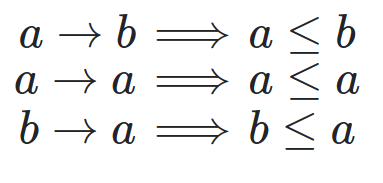All these arrows can hold, and there is a loop between a and b

### Partial order

Pre-order without loops.

Example: “less” order relation.

### Total order

All objects have relations

## Monoid

Category with 1 object and many morphisms from that object to itself.

Hom-set: M(m, m) — set of all arrows.

## Terminal and initial objects

Terminal object — object to which there is arrow from any other object in category.

Initial object — object from which there is an arrow to any other object in category.

Opposite category is a category with “reversed arrows”. Terminal object is initial in opposite category.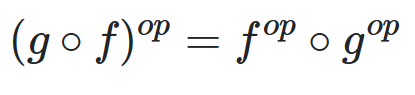## Product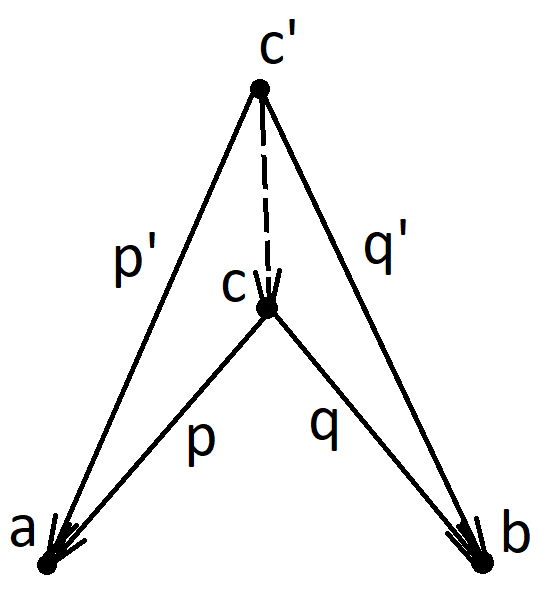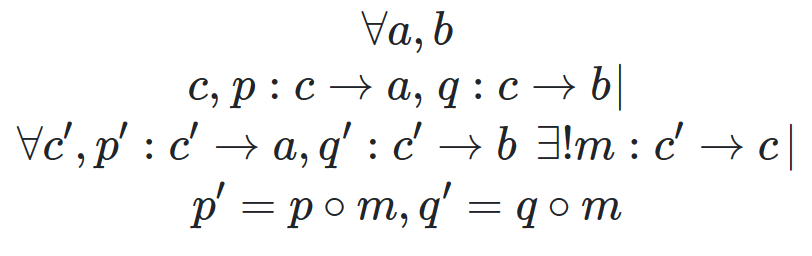c is a better product than c’ (in terms of order relation)

p, q — projections

Product in programming is a Pair:

``````data class Pair<A : Any, B : Any>(
val fst: A,
val snd: B
)
``````

## Sum (Co-Product)

Similar to Product, with reversing arrows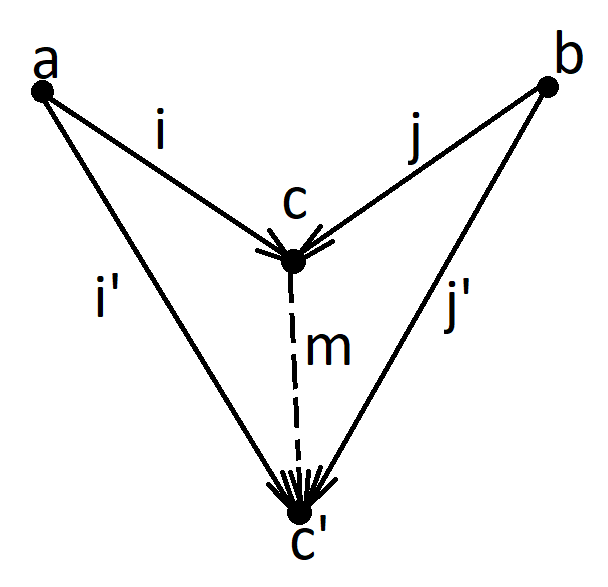i, j — injections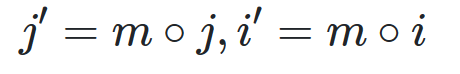In programming it can be described as Either:

``````class Either<A: Any, B: Any>
private constructor(private val a: A?, private val b: B?) {

companion object {

fun <A: Any, B: Any> left(a: A): Either<A, B> {
return Either(a, null)
}

fun <A: Any, B: Any> right(b: B): Either<A, B> {
return Either(null, b)
}
}
}
``````

## Algebraic Data Types

### Product

• symmetry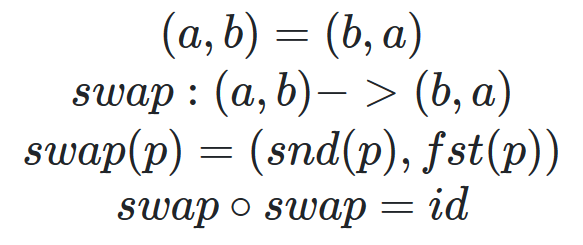• associativity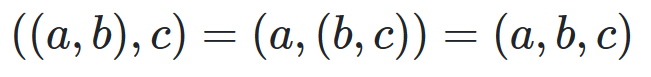• identity### Sum

• symmetry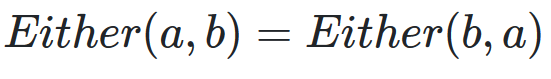• associativity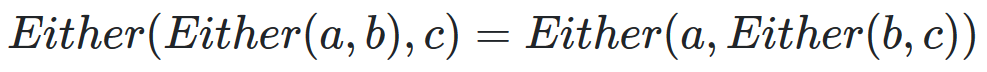• identity### Product and Sum

• distribution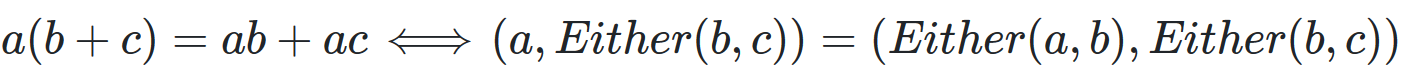• annihilation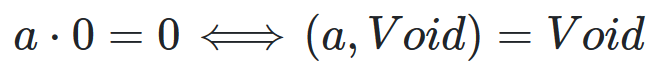### Semiring

With defined product and sum (without inverse operations) we get Semiring.

### Example 1 Boolean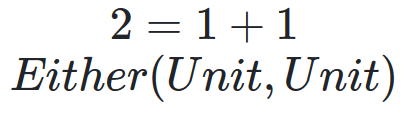### Example 2 Option### Example 3 List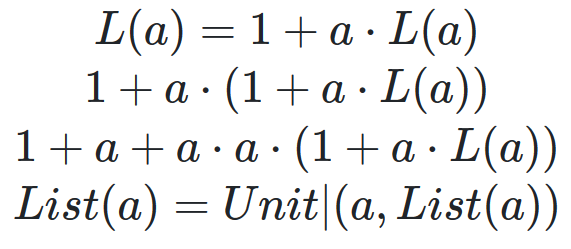## Functor

Functor — mapping from one category to another with preserving structure. Objects are mapped into objects, morphisms into morphisms. Preserving structure means that composition and identity is preserved.

Functor can be though as a container.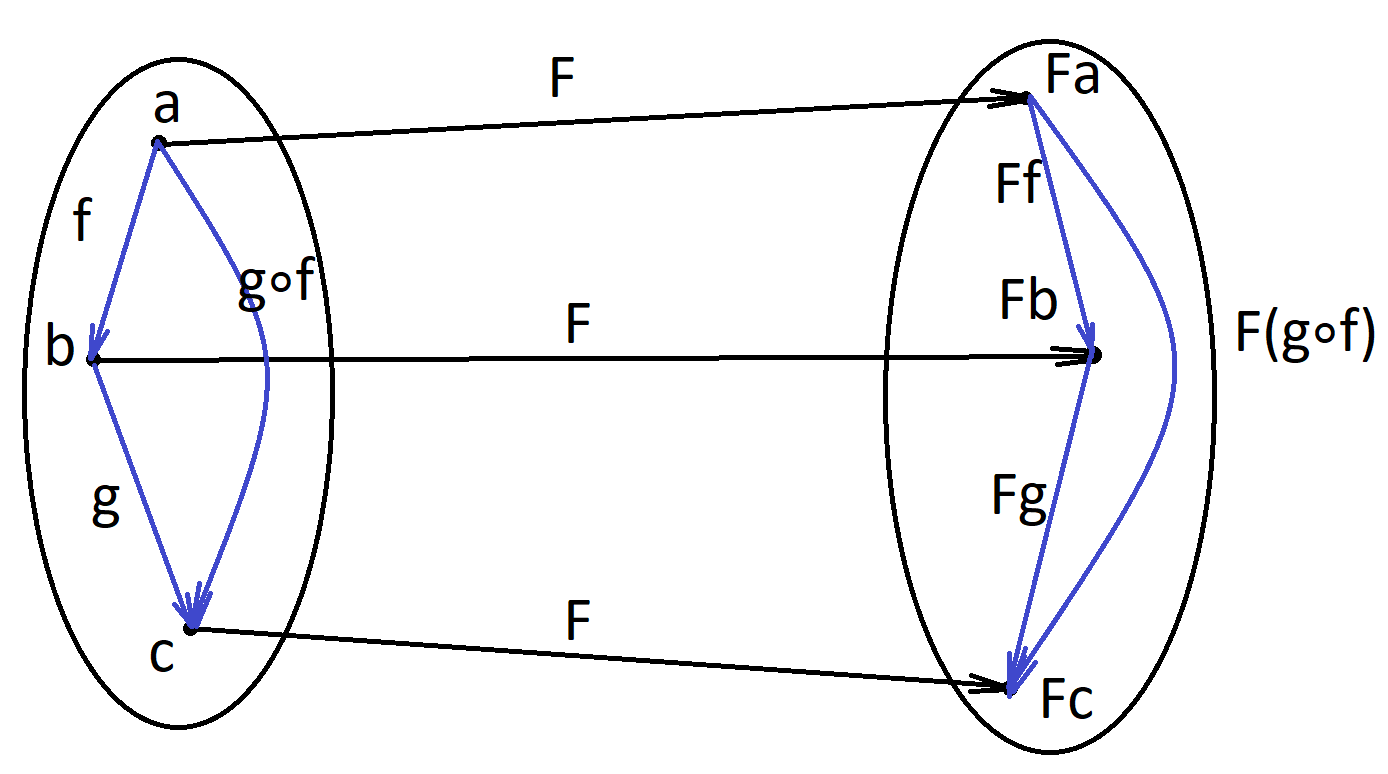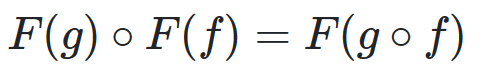### Special Types of Functors

• Faithful — injective on all hom-sets

• Full — surjective on all hom-sets

• Constant Δc — functor which maps all objects into single object c and all morphisms into single morphism idc

• Endofunctor — functor from one category to the same category

### Example 1 Option

• mapping objects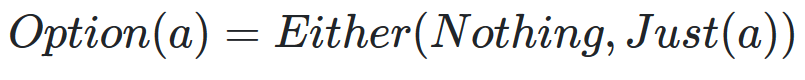• mapping morphisms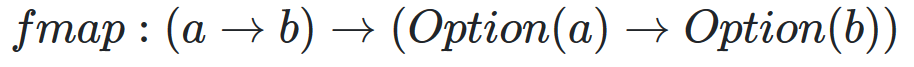• preserve identity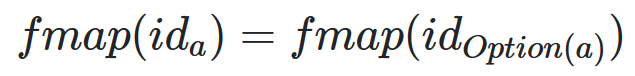• preserve composition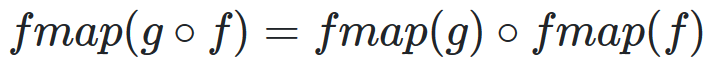### Example 2 List

• mapping objects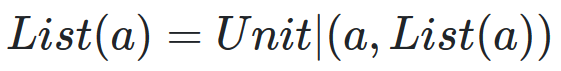### Functor in programming

``````interface Functor<A> {
fun <B> map(f: (A) -> B): Functor<B>
}
``````

## BiFunctor

### Cat

Cat — category of categories

• objects are categories

• morphisms are functors

### Product Category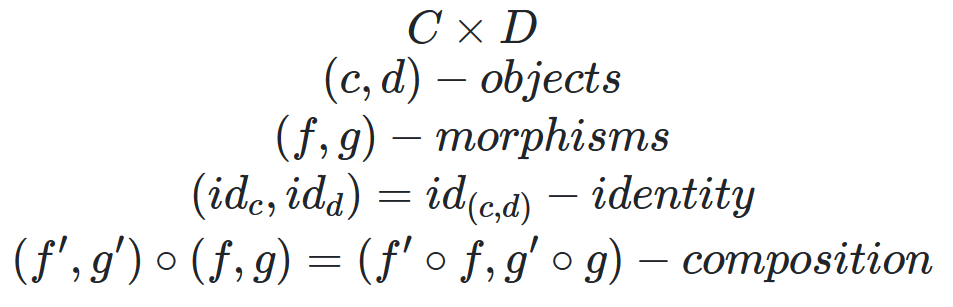### BiFunctor

BiFunctor is mapping from product category into another category.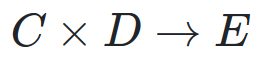Sum is also a BiFunctor

### BiFunctor in programming

``````interface Bifunctor<A : Any, B: Any> {

fun <C : Any> first(f: (A) -> C): Bifunctor<C, B>

fun <D : Any> second(f: (B) -> D): Bifunctor<A, D>

fun <C : Any, D : Any> bimap(f: (A) -> C, g: (B) -> D): Bifunctor<C, D>
}
``````

## ProFunctor

Constant Functor

``````data class Const<C : Any, A : Any>(val c: C): Functor<A> {

override fun <B : Any> fmap(f: (A) -> B): Const<C, B> {
return Const<C, B>(c)
}
}
``````

Identity Functor

``````data class Just<A : Any>(val a: A) : Functor<A> {

override fun <B : Any> fmap(f: (A) -> B): Just<B> {
return Just(f(a))
}
}
``````

Maybe via composition

``````class Maybe<A> = Either(Const<Unit, A>, Identity<A>)
``````

Either is a BiFunctor, Const and Identity are Functors.

### ProFunctor

ProFunctor — mapping from product of category with its opposite category to that category.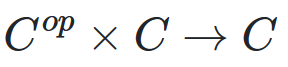``````interface Contravariant<A : Any> {

fun <B : Any> contramap(f: (B) -> A): Contravariant<B>
}
``````

### ProFunctor in programming

``````interface Profunctor<A : Any, B : Any> {

fun <C : Any> lmap(f: (C) -> A): Profunctor<C, B>

fun <D : Any> rmap(f: (B) -> D): Profunctor<A, D>

fun <C : Any, D : Any> dimap(f: (C) -> A, g: (B) -> D): Profunctor<C, D>
}
``````

## Functions/exponentials

### Currying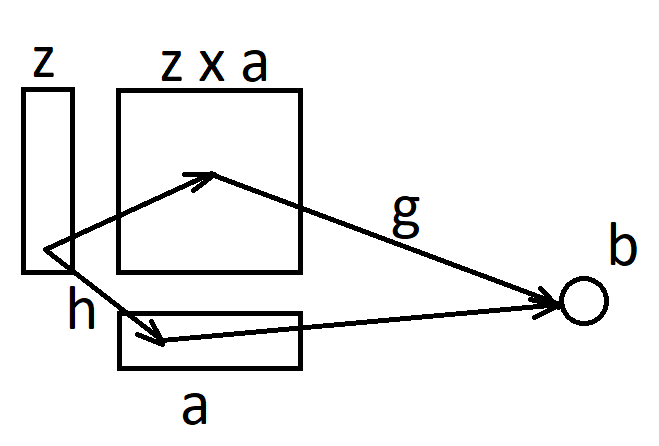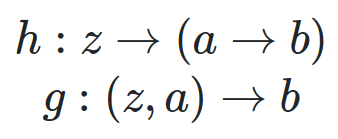``````fun <A, B, C> curry(f: (Pair<A, B>) -> C): (A) -> ((B) -> C)
fun <A, B, C> uncurry(f: (A) -> ((B) -> C)): (Pair<A, B>) -> C
``````

### Cartesian Closed Category (CCC)

Category is CCC if it has:

• product

• exponential

• terminal object

### Exponential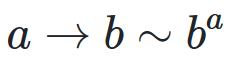### Examples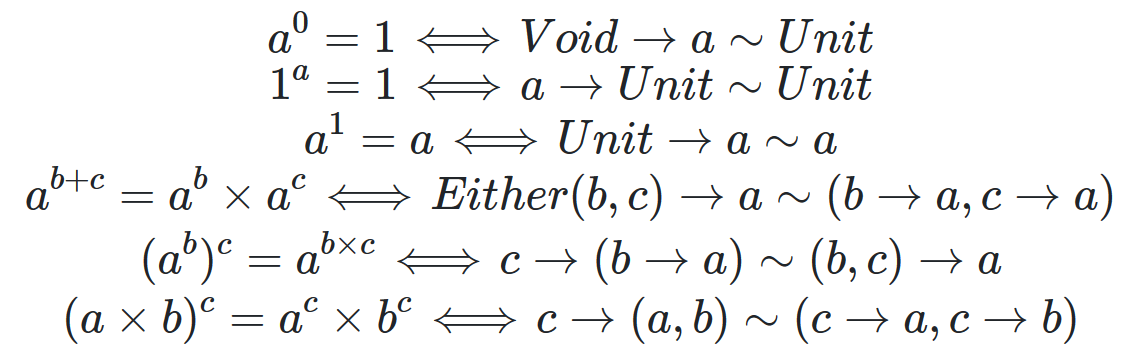### Proposition of types / Curry-Howard-Lambok isomorphism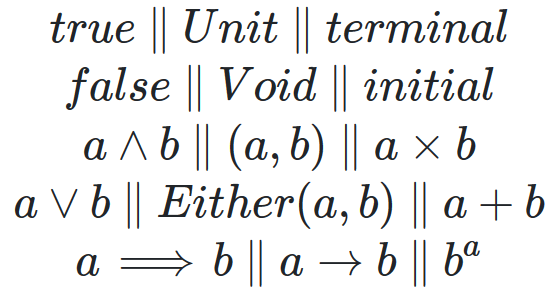## Natural transformation

Natural transformation — mapping between Functors (or objects to morphisms).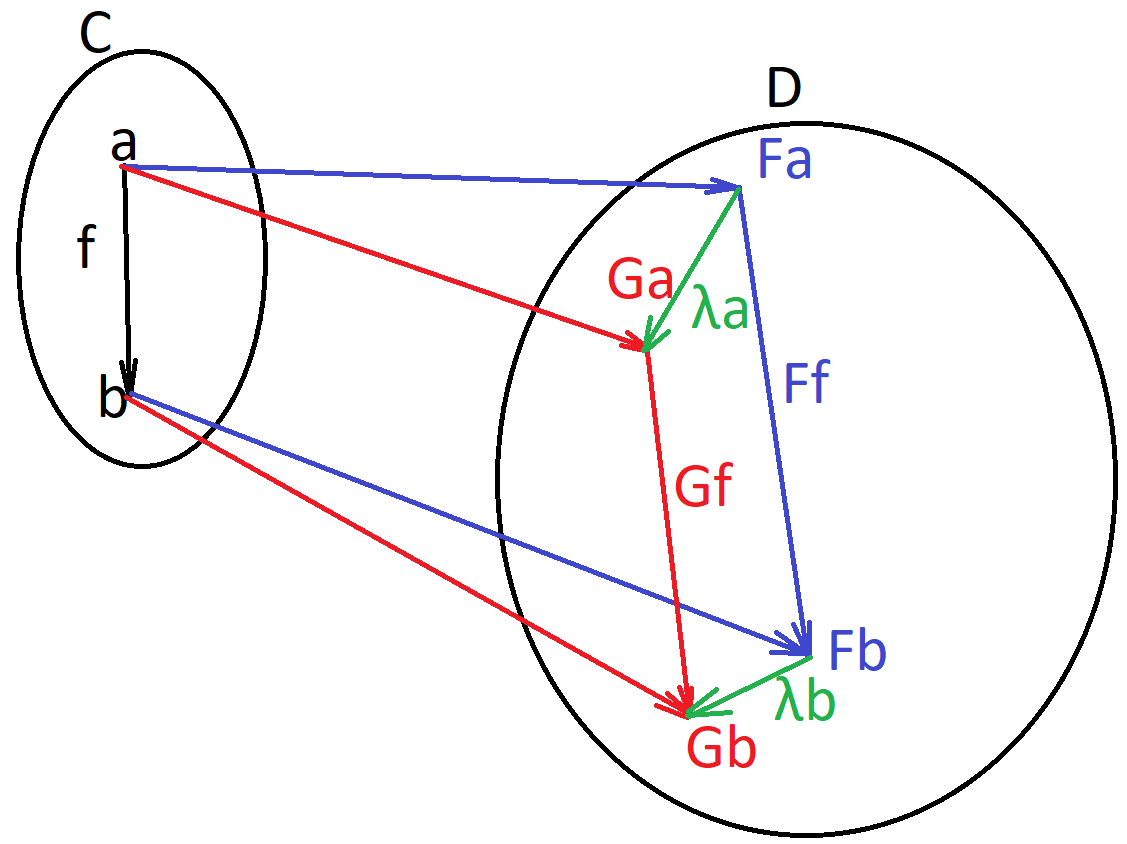Naturality square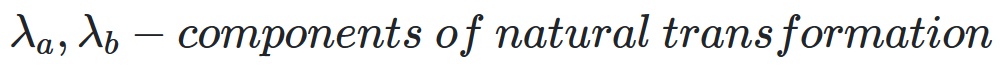Natural transformation is isomorphic if all components are isomorphic.

### Naturatlity condition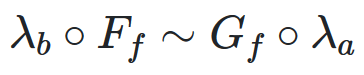### Natural transformation in programming

NT in programming is polymorphic function.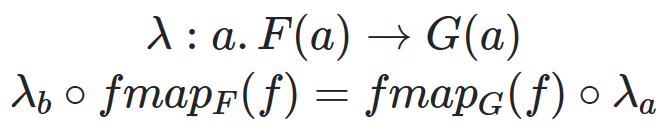### Example

``````fun <A> List<A>.head(): Option<A> {
return if (this.isEmpty()) None else Just(this.first())
}

``````

Reversing order of function application can be used in optimizations.

### Intuition

Functor — map container contents

Natural transformation — map container

Naturality condition says that it doesn’t matter what to do first and what second: map container or map container contents.

### Examples of Natural Transformations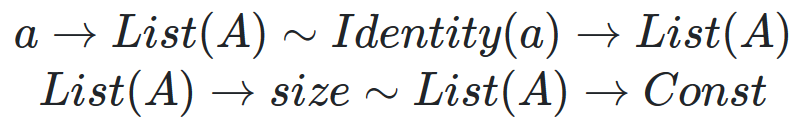Monad — Monoid in category of endofunctorsMonoid

Monoid object in category [C, C] (category of endofunctors) is a Monad

### Applicative

Applicative is a functor with: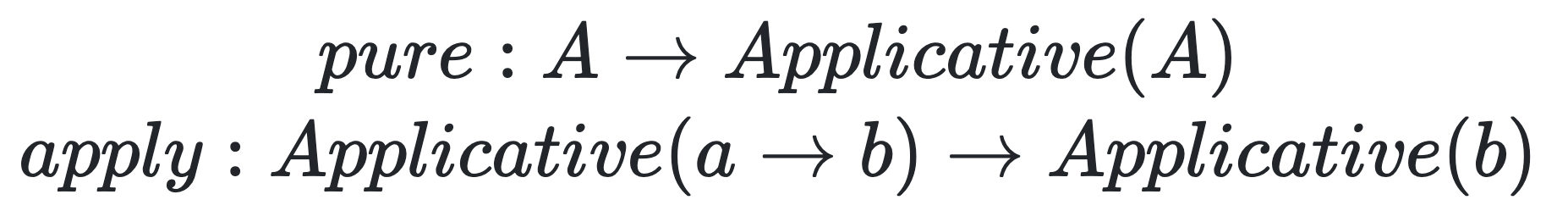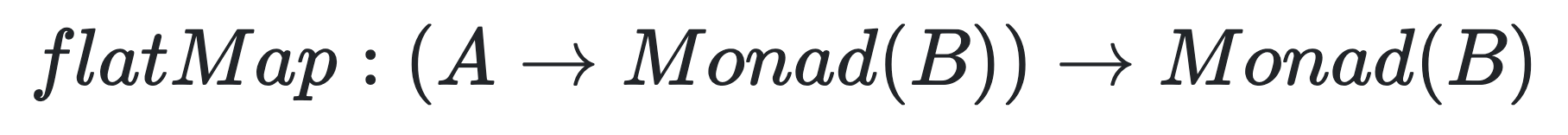Other functions: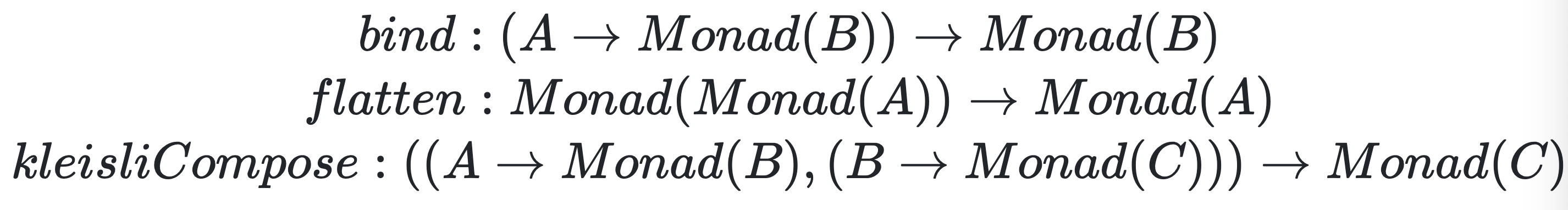## Final words

Lectures were great, a lot of insides on the how world is constructed, how other math disciplines are based on these very high-level thoughts. And how all of this is related to programming, which is for sure a computer science.

To make a bit of a practice made few implementations of things which were an examples throughout the course. For sure, implementation is not that great, but it is in Kotlin which is more functional than Java (but not that as Scala or Haskell). Implementations are just examples and have no real usage. If one is interested in better implementations I think it is good to take a look at arrow-kt.

Happy coding